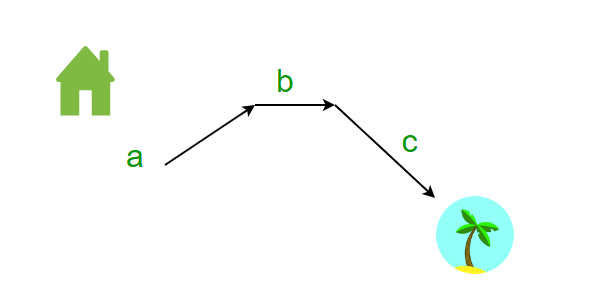Related Articles

# Puzzle 68 | Walking down the hill

• Difficulty Level : Easy
• Last Updated : 26 Aug, 2021

I can walk 3 miles per hour uphill and 4 miles per hour on flat land and 6 miles per hour downhill.If I reached beach near by me, walking for 50 minutes and I walked back home in 1 hour, can you calculate the total distance from my house to the beach? (Note: I walk with same uniform speed.)Solution: This might seem like an incomplete data problem, but it is not.

Let us assume that I walk in total from my home to the beach

a mile’s uphill
b miles on flat land
c miles’ downhill
The total distance is a+b+c

When walking from beach to my home, uphill will be downhill and vice versa

Therefore,

c miles’ uphill
b miles on flat land
a mile’s downhill
The total distance is a+b+c

distance/speed =  time

By solving the above two, we get two equations

a/3 + b/4 + c/6 = 50/60 hours
c/3 + b/4 + a/6 = 1 hour
=>    2a + 3b + 4c = 12

2c + 3b + 4a = 10

Now we need the distance a + b + c

By adding the above two equations, we get

6a + 6b + 6c = 22

Therefore   a + b + c =  22/6 =  11/3 miles or 3 and 2/3 miles